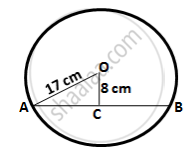Share

# The Radius of a Circle is 17.0 Cm and the Length of Perpendicular Drawn from Its Centre to a Chord is 8.0 Cm. Calculate the Length of the Chord. - Mathematics

Course

#### Question

The radius of a circle is 17.0 cm and the length of perpendicular drawn from its centre to a chord is 8.0 cm. Calculate the length of the chord.

#### Solution

Let AB be the chord and O be the centre of the circle.
Let OC be the perpendicular drawn from O to AB.We know, that the perpendicular to a chord, from the centre of a circle, bisects the chord.
∴ AC = CB
In ∆OCA,
OA2 = OC2 + AC2  (By Pythagoras theorem)
⇒ AC2 = (17)2 - (8)2 = 225
⇒ AC = 51 cm
∴ AB = 2 AC = 2 × 15 = 30 cm

Is there an error in this question or solution?